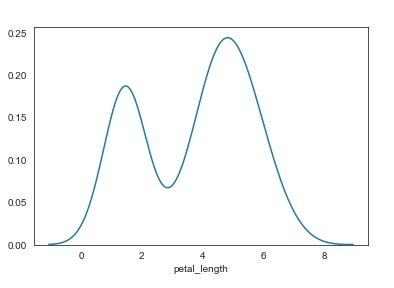# How can Seaborn library be used to display kernel density estimations in Python?

Visualizing data is an important step since it helps understand what is going on in the data without actually looking at the numbers and performing complicated computations. Seaborn is a library that helps in visualizing data. It comes with customized themes and a high-level interface.

Kernel Density Estimation, also known as KDE is a method in which the probability density function of a continuous random variable can be estimated.

This method is used for the analysis of the non-parametric values. While using ‘distplot’, if the argument ‘kde’ is set to True and ‘hist’ is set to False, the KDE can be visualized.

Let us see how we can visualize a kernel density estimation in Python −

## Example

import pandas as pd
import seaborn as sb
from matplotlib import pyplot as plt
sb.distplot(df['petal_length'],kde = True, hist = False)
plt.show()

## Output## Explanation

• The required packages are imported.
• The input data is ‘iris_data’ which is loaded from the scikit learn library.
• This data is visualized using the ‘distplot’ function.
• Here, the parameter ‘kde’ is set to true since we only want to display the histogram.
• This visual data is displayed on the console.

Note − When the value of ‘kde’ is specified as False, only the histogram is displayed.

Updated on: 11-Dec-2020

51 Views